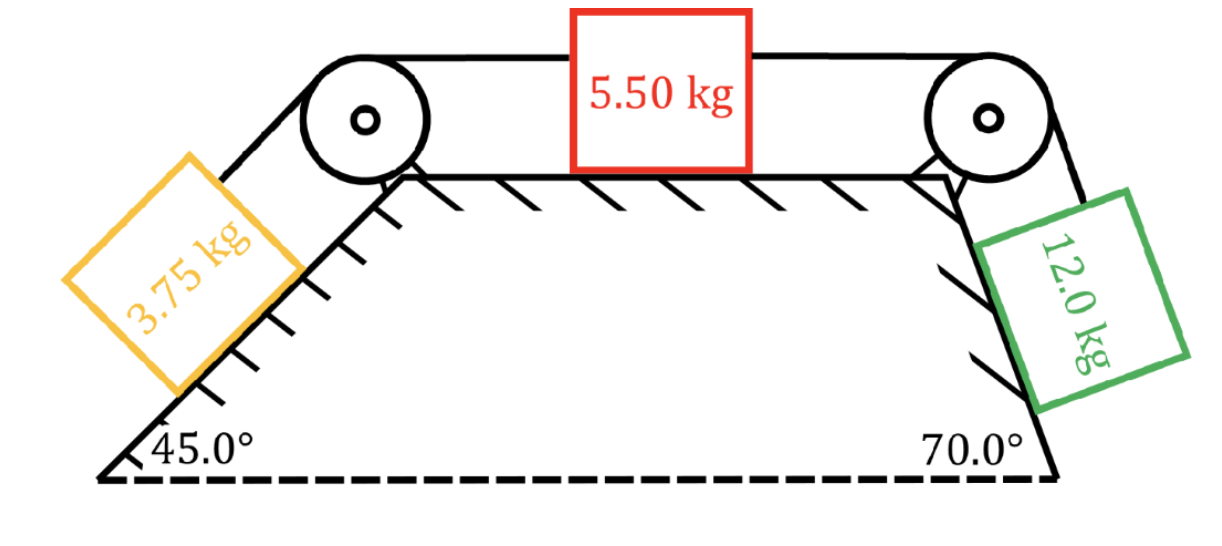# Tension and pulley problems

### Tension and pulley problems

#### Lessons

In this lesson, we will learn:
• What is tension?
• How to calculate tension
• Problem solving with tension

Notes:

• Tension is the force of a rope (or string, cable, etc.) pulling on an object.
• Tension is always a pulling force: a rope can't push!
• There is no formula for tension. Tension force acting on an object must be calculated from Newtons' second law.
• If the rope is assumed to be massless and non-stretchy, then the pulling force at either end of the rope must be equal in magnitude.
Newton's Second Law

$\Sigma \vec{F} = \vec{F}_{net} = m\vec{a}$

$\Sigma \vec{F}:$ sum of all forces, in newtons (N)

$\vec{F}_{net}:$ net force, in newtons (N)

$m:$ mass, in kilograms (kg)

$\vec{a}:$ acceleration, in meters per second squared $(m/s^{2})$

Newton's Third Law

For object A exerting a force on object B:

$\vec{F}_{A on B} = - \vec{F}_{B on A}$

$\vec{F}_{A on B}:$ force A is exerting on B, in newtons (N)

$\vec{F}_{B on A}:$ force B is exerting on A, in newtons (N)

Atwood Machine Equation

$a = g\frac{(m_{1}-m_{2})}{(m_{1}+m_{2})}$

$a:$ acceleration of masses, in meters per second squared $(m/s^{2})$

$g:$ acceleration due to gravity, in meters per second squared ($m/s^{2}$)

$m_{1}:$ mass of first hanging mass, in kilograms (kg)

$m_{2}:$ mass of second hanging mass, in kilograms (kg)

• Introduction
Introduction to tension:
a)
What is tension? How to calculate tension using Newton's second law.

b)
Tension force at either end of a rope

• 1.
Calculating tension using Newton's second law

A train toy is made up of three carts of different masses connected by pieces of string. If string A is pulled with 7.05 N [right], find the tension in strings A, B, and C.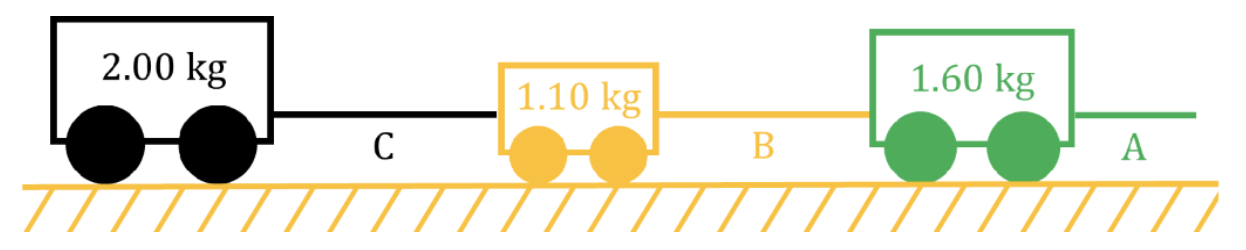• 2.
Solving vertical pulley problems (Atwood machine problems)

Two boxes (5.00 kg and 7.25 kg) are tied together by a rope and hang vertically from a frictionless pulley. What is the acceleration of each box, and the tension in the rope?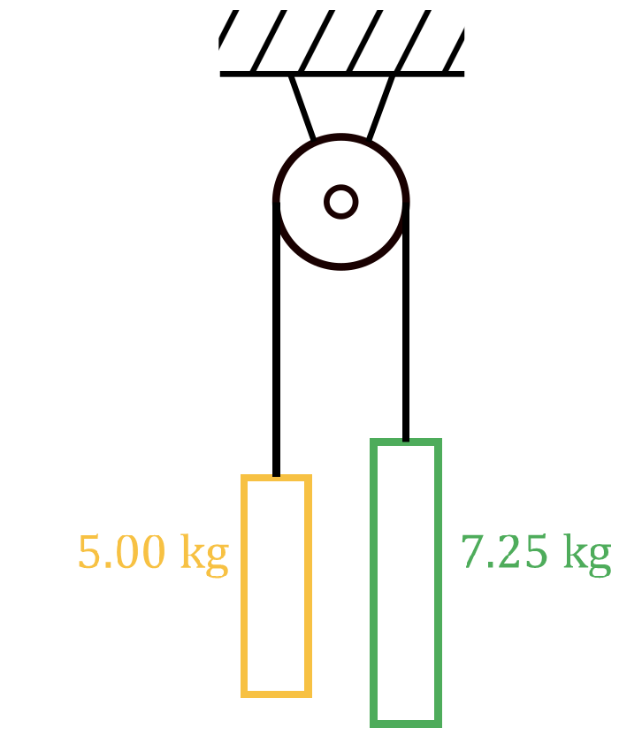• 3.
Solving horizontal pulley problems with friction

Two boxes (8.00 kg and 4.40 kg) are tied together by a rope and hang from a pulley as shown. The coefficient of friction between the ground and the 8.00 kg box is 0.250.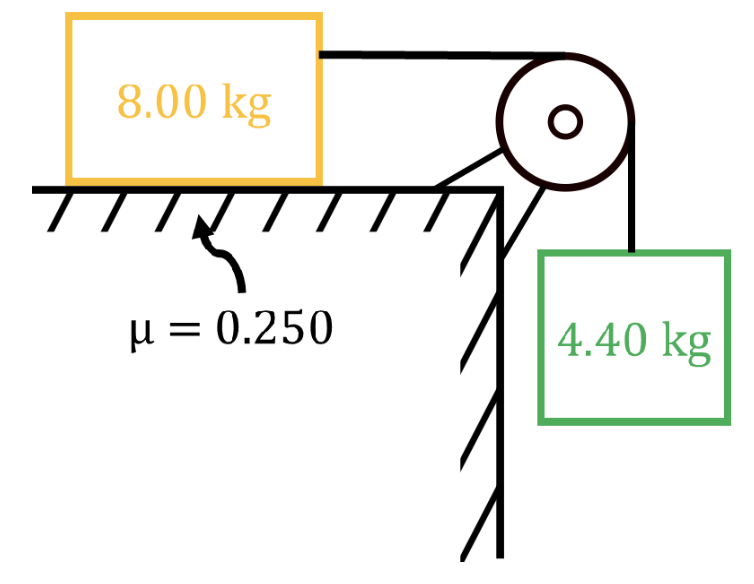a)
Find the acceleration of the boxes by treating the two boxes as separate objects.

b)
Find the acceleration of the boxes by treating the two boxes two boxes as one object ("black box" method).

c)
Find the tension in the rope.

• 4.
Slope with friction pulley problem using the "black box" method

Two boxes (3.50 kg and 2.00 kg) are tied together by a rope and hang from a pulley as shown. The coefficient of friction between the ground and the 3.50 kg box is 0.150. What is the acceleration of each box, and the tension in the rope?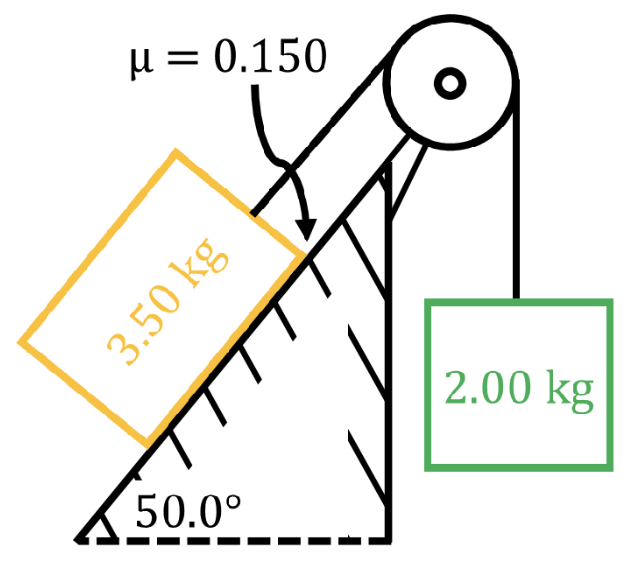• 5.
Three box pulley problem with slopes and friction using the "black box" method

Three boxes (3.75 kg, 5.50 kg and 12.0 kg) are tied together by two ropes and hang from a pulley as shown. The coefficient of friction between the ground and the boxes is 0.250. What is the acceleration of each box, and the tension in ropes A and B?